# Regular semi-group

(diff) ← Older revision | Latest revision (diff) | Newer revision → (diff)

A semi-group in which every element is regular (see Regular element).

An arbitrary regular semi-groupcontains idempotents (see Idempotent), and the structure ofis determined to a considerable extent by the "structure" and the "distribution" inof the setof all its idempotents (cf. Idempotents, semi-group of). Regular semi-groups with a unique idempotent are just groups. In the first place,can be regarded as a partially ordered set in a natural way. There are known structure theorems describing a regular semi-groupwith certain natural restrictions on the set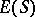. One such restriction (for semi-groups with zero) is that all non-zero idempotents are primitive (see Completely-simple semi-group); a semi-group with this property is called primitive. The following conditions on a semi-groupare equivalent: a)is a primitive regular semi-group; b)is a regular semi-group equal to the union of its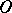-minimal (right) ideals (see Minimal ideal); and c)is an-direct union of completely-simple semi-groups. The structure of regular semi-groups is also known in the case whenis a chain with the order type of the negative integers .

A more informative view ofis obtained if one defines a partial operationon it in the following way. If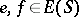are such that at least one of the products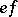,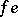is equal to eitheror, then; one then sets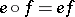. The resulting partial algebra can be axiomatized in terms of two quasi-order relationsand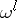. These are closely related to the given partial operation (the realization of these relations inis as follows: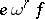means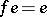,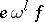means; then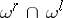is the natural partial order on). Such a partial algebra is called a bi-ordered set (see ). An arbitrary regular semi-group can be constructed in a specific way from a bi-ordered set and groups. It is thus possible to classify regular semi-groups in terms of bi-ordered sets. Among the types of semi-groups that have been investigated in this way are combinatorial regular semi-groups (see ), that is, those whose only subgroups consist of one element.

A homomorphic image of a regular semi-group is regular. Every normal complex of a regular semi-group which is a sub-semi-group contains an idempotent. An arbitrary congruence (cf. Congruence (in algebra)) on a regular semi-group is uniquely determined by its classes that contain idempotents. A congruence on a regular semi-groupseparates idempotents if and only if it is contained in the relation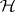(see Green equivalence relations). The set of such congruences forms a modular sublattice with a zero and a unit element in the lattice of all congruences on(cf. also Modular lattice). A regular semi-group is called fundamental if this sublattice contains only the equality relation. Every combinatorial regular semi-group is fundamental. Fundamental regular semi-groups are important, not only as one of the more visible types of regular semi-groups, but also because of their "universality" property in the class of all semi-groups. More precisely, for any bi-ordered setit is possible to construct in a canonical way a fundamental regular semi-group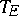such thatis the bi-ordered set of all idempotents, and for any regular semi-groupwith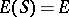there is a homomorphism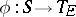that separates idempotents and is such thatis a sub-semi-group ofcontaining(for various constructions of, see , , , ). A regular semi-groupis fundamental if and only ifis injective.

Ifis a regular semi-group, then the sub-semi-group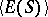generated by its idempotents is also regular. The sub-semi-groupexerts an essential influence on the structure of. A regular semi-group is idempotently generated if and only if the same is true for each of its principal factors . In an idempotently-generated regular semi-group, any elementcan be written in the form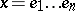, whereand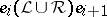for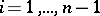(here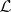andare Green equivalence relations, ). A sequence of idempotentswith the above property is called an-chain. In a bi-simple idempotently-generated semi-group, any two idempotents are connected by an-chain, and if they are comparable in the sense of the natural partial order, then such a chain has length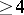.

If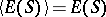, that is, the product of any two idempotents is again an idempotent, then the regular semi-groupis called orthodox. The class of orthodox semi-groups contains, in particular, all inverse semi-groups. A semi-group is orthodox if and only if its principal factors are. There are structure theorems for orthodox semi-groups (see , ).

The natural partial order oncan be extended to the regular semi-groupin the following way:if there are idempotentsandsuch that. Ifis inverse, the relation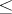becomes the natural partial order, and it is also called the natural partial order for an arbitrary regular semi-group. The relationon the regular semi-groupis compatible with the multiplication if and only if, for any idempotent, the sub-semi-group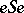is inverse  (cf. Inversion semi-group). Regular semi-groups with this property are called pseudo-inverse. A wider class is formed by pseudo-orthodox semi-groups (those in which the sub-semi-groupis orthodox for any idempotent). These classes of semi-groups are also called "locally inverse regular semi-grouplocally inverse" and "locally orthodox regular semi-grouplocally orthodox" , respectively. A regular semi-group is called natural if the set of all its group elements (see Regular element) is a sub-semi-group. There are structure theorems for pseudo-inverse, pseudo-orthodox  and natural  regular semi-groups.

Numerous structure theorems for various types of regular semi-groups represent (sometimes very remote) generalizations and modifications of the structure of a Rees semi-group of matrix type or of the sum of the direct spectrum of groups (see Clifford semi-group), and are based on various representations of semi-groups and their decomposition into subdirect products (see , ). See also Semi-group.

How to Cite This Entry:
Regular semi-group. Encyclopedia of Mathematics. URL: http://encyclopediaofmath.org/index.php?title=Regular_semi-group&oldid=13668
This article was adapted from an original article by L.N. Shevrin (originator), which appeared in Encyclopedia of Mathematics - ISBN 1402006098. See original article